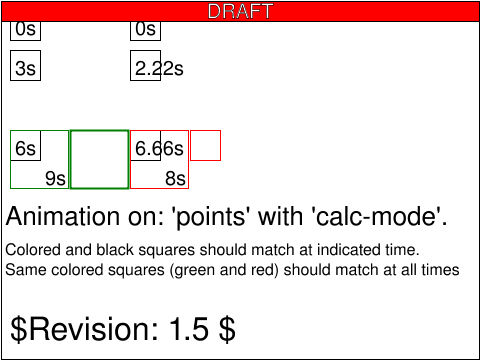Tests that contain the draft-watermark are under development and may be incorrectly testing a feature.

# animate-elem-53-t.svg## Operator Script

1. The green squares should animate together side by side. This applies to the blue ones as well. 2. The time values indicate when the squares should reach the corresponding reference square. 3. The total distance is 0+40+80+24.14=144.14 a. The "green animation" is 9 sec and linear so each interval should get 3 sec. b. The "blue animation" is 8 sec and paced so the intervals should get 2.22, 4.44 and 1.34 sec each.

Here comes a more detailed description of the animation. The left green square (LG) is animated by animating the points with a value array, consisting of 4 lists of points. This is an animation with calc-mode=linear so an equal amount of time should be spent on all 4 intervals. The right green square (RG) is animated by a simple linear motion followed by a scale to follow LG. The last scale by 1.9428 correspond to a movement of the lover right corner of the square by sqrt((30*0.9428)^2 + (30*0.9428)^2) which is approximately 40 distance units. This is the same distance as the first interval in the values array (and half the second interval). The length (in terms of distance) is not really important for the green squares but for the blue squares which are animated with calc-mode=paced the length is used to calculate the time for each interval. Since the first and last interval are of the same length which totals to the length of the middle interval, the interval should be given time according to [27.75%(2.22sec);55.5%(4.44sec);16.75%(1.34sec)]. So the left blue square (LR) is animated just as the LG square but with calc-mode=paced. The same applies to the right blue square (RR) that has default calc-mode (paced for animateMotion) compablue to the RG square that has calc-mode=linear. The calc-mode for the scale of RR (and RG) is not important since it's not a value list type of animation.

## Pass Criteria

[[Describe the pass criteria of the test here. The pass criteria is what should be displayed when the test is run.]]

## Test Description

The purpose of this test is to test animation of points and calcmode.